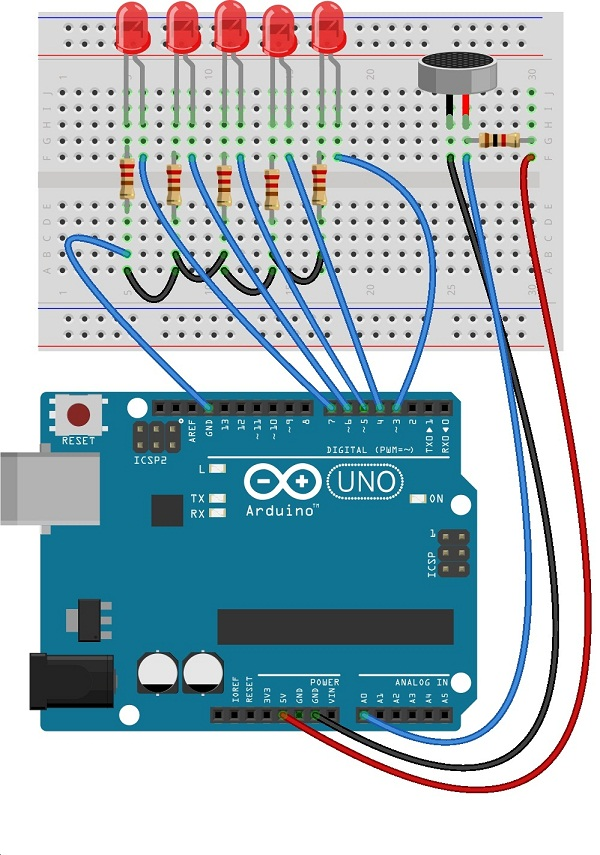This project gives visual output for different level of analog input. Here the condenser mic acts as analog input sensor. You can use any sensor which you want visual bar graph output.

The mic biased with power supply from Arduino +5V pin and ground pin, array of LEDs are connected with digital pins of Arduino.Circuit diagram[code]

const int ledCount = 5;
const int ledPins[] = {3, 4, 5, 6, 7};

const int analogPin = A0; // Analog input pin connected to the variable resistor

void setup() {

for(int thisLed = 0; thisLed < ledCount; thisLed++)
{
pinMode(ledPins[thisLed], OUTPUT);
}
}

void loop() {

int ledLevel = map(sensorReading, 0, 1023, 0,ledCount);
for(int thisLed = 0; thisLed < ledCount; thisLed++)
{
if (thisLed < ledLevel)
{
digitalWrite(ledPins[thisLed], HIGH);
}
else
{
digitalWrite(ledPins[thisLed], LOW);
}
}
}

[/code]

Components List

 S.No Name Quantity 1. Arduino uno 1 2. LED Red 5 3. Resistor-220Ω Resistor 1KΩ 5 1 4. Connecting wires as required 5. Mic 1

Prototype